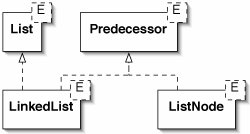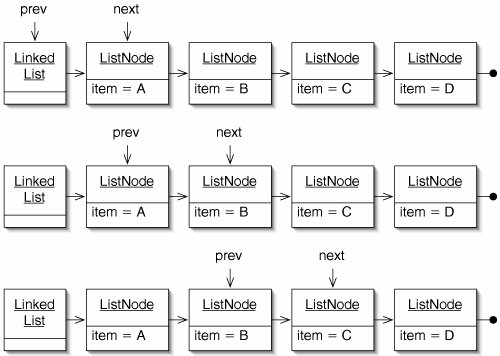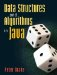We now present a class LinkedList which implements the List interface from Chapter 5. A LinkedList has one field front, which is a ListNode. As in a LinkedStack, this node may in turn contain other ListNodes. The fields and trivial methods are shown in Figure 6-18.

Figure 6-18. A LinkedList contains a single chain of ListNodes.

 ``` 1 /** A linked list. */ 2 public class LinkedList implements List { 3 4 /** The first node in the List. */ 5 private ListNode front; 6 7 /** A LinkedList is initially empty. */ 8 public LinkedList() { 9 front = null; 10 } 11 12 public boolean isEmpty() { 13 return front == null; 14 } 15 16 } ```

Many of the LinkedList methods involve walking down the chain of references. The general form of this code is:

```for (ListNode node = front;
node != null;
node = node.getNext()) {
// Do something with node.getItem()
}
```

The variable node refers to the node currently being processed. To advance to the next node, we set node to node.getNext().

This technique is used in the contains(), size(), and toString() methods (Figure 6-19).

Figure 6-19. Many LinkedList methods walk down the list using for loops.

 ``` 1 public boolean contains(E target) { 2 for (ListNode node = front; 3 node != null; 4 node = node.getNext()) { 5 if (node.getItem().equals(target)) { 6 return true; 7 } 8 } 9 return false; 10 } 11 12 public int size() { 13 int tally = 0; 14 for (ListNode node = front; 15 node != null; 16 node = node.getNext()) { 17 tally++; 18 } 19 return tally; 20 } 21 22 public String toString() { 23 String result = "("; 24 for (ListNode node = front; 25 node != null; 26 node = node.getNext()) { 27 result += node.getItem() + " "; 28 } 29 return result + ")"; 30 } ```

Notice that these loops do not use an int to keep track of the current position in the list. We know when we've reached the end of the loop when node is null. In contrast, the get() and set() methods (Figure 6-20) do use an int, since they want to advance only a specific number of nodes.

Figure 6-20. The get() and set() methods advance index nodes down the list.

 ``` 1 public E get(int index) { 2 ListNode node = front; 3 for (int i = 0; i < index; i++) { 4 node = node.getNext(); 5 } 6 return node.getItem(); 7 } 8 9 public void set(int index, E target) { 10 ListNode node = front; 11 for (int i = 0; i < index; i++) { 12 node = node.getNext(); 13 } 14 node.setItem(target); 15 } ```

It appears that the add() method can be written in a similar way. We advance down the List until we get to the last node, at which point we tack on a new one (Figure 6-21).

Figure 6-21. Broken first draft of the add() method.

 ```1 public void add(E target) { 2 ListNode last = front; 3 while (last.getNext() != null) { 4 last = last.getNext(); 5 } 6 last.setNext(new ListNode(target)); 7 } ```

Unfortunately, there is a catch. What if the List is empty? The method will throw a NullPointer-Exception when we try to invoke last.getNext() on line 4.

One solution is to add code to deal with this special case (Figure 6-22). There is, however, a more elegant way to handle the problem.

Figure 6-22. Special code is needed to handle the case where the LinkedList is empty.

 ``` 1 public void add(E target) { 2 if (isEmpty()) { 3 front = new ListNode(target); 4 } else { 5 ListNode last = front; 6 while (last.getNext() != null) { 7 last = last.getNext(); 8 } 9 last.setNext(new ListNode(target)); 10 } 11 } ```

The Predecessor Interface

Lines 3 and 9 of Figure 6-22 do almost exactly the same thing: create a new ListNode and set some reference to point to it. In line 3, this reference is the front field of a LinkedList. In line 9, it is the next field of a ListNode.

We can use polymorphism to write a single line of code which does whichever of these things is appropriate. If we have a variable which can refer to either a LinkedList or a ListNode, we can invoke a method on it which says, "Here is a new node. Make it the next one after you."

This variable must be of a polymorphic type. We have two choices: it can be some class which is a superclass of both LinkedList and ListNode, or it can be an interface which both of these classes implement. Since these classes have no fields or methods in common, a superclass doesn't really make sense; an interface is a better choice.

The Predecessor interface is given in Figure 6-23. It also describes a method getNext(), which returns the next node after the Predecessor.

Figure 6-23. The Predecessor interface.

 ``` 1 /** 2 * Something that has a next ListNode. 3 */ 4 public interface Predecessor { 5 6 /** Get the next ListNode. */ 7 public ListNode getNext(); 8 9 /** Set the next ListNode. */ 10 public void setNext(ListNode next); 11 12 } ```

In order to use this interface, both ListNode and LinkedList will have to implement it. ListNode already provides these methods, so we just have to change the first noncomment line to:

```public class ListNode implements Predecessor {
```

In the LinkedList class, we have to provide both of these methods and change the first noncomment line (Figure 6-24).

Figure 6-24. The LinkedList class must be modified to implement Predecessor.

 ``` 1 /** A linked list. */ 2 public class LinkedList implements List, Predecessor { 3 4 public ListNode getNext() { 5 return front; 6 } 7 8 public void setNext(ListNode next) { 9 front = next; 10 } 11 12 } ```

The LinkedList class now implements two different interfaces. This is illustrated in Figure 6-25.

Figure 6-25. The Predecessor interface is implemented by two classes. The LinkedList class implements two interfaces.Now we can write a more elegant version of the add() method for LinkedList (Figure 6-26).

Figure 6-26. The Predecessor interface allows us to write a shorter version of add() than the one in Figure 6-22.

 ```1 public void add(E target) { 2 Predecessor last = this; 3 while (last.getNext() != null) { 4 last = last.getNext(); 5 } 6 last.setNext(new ListNode(target)); 7 } ```

Two-Finger Algorithms

We now consider the two remove() methods. One of these methods removes the element at a particular position; the other removes a particular Object. Both make use of the technique of splicing out a node. They also use the Predecessor interface to avoid special code for the case where the node being removed is the first one.

In each method, we walk down the list looking for either the ith node or the node containing target. Once we find the offending node, we have a problem: we've forgotten the previous node!

Our solution is to keep track of two nodes: the previous one and the current one (Figure 6-27). Since such an algorithm points at two consecutive nodes on each pass through its loop, it is known as a two-finger algorithm.

Figure 6-27. In a two-finger algorithm, two variables (prev and next) refer to two consecutive ListNodes. Labels on the references between objects are omitted for brevity.The code for the remove() methods is given in Figure 6-28.

Figure 6-28. Both of the remove() methods are two-finger algorithms.

 ``` 1 public E remove(int index) { 2 Predecessor prev = this; 3 ListNode node = front; 4 for (int i = 0; i < index; i++) { ``` 5 prev = node; 6 node = node.getNext(); 7 } 8 prev.setNext(node.getNext()); 9 return node.getItem(); 10 } 11 12 public boolean remove(E target) { 13 Predecessor prev = this; 14 ListNode node = front; 15 while (node != null) { 16 if (node.getItem().equals(target)) { 17 prev.setNext(node.getNext()); 18 return true; 19 } 20 prev = node; 21 node = node.getNext(); 22 } 23 return false; 24 }

The ListIterator Class

All that remains is the iterator() method. As in ArrayList, it simply creates and returns an Iterator (Figure 6-29).

Figure 6-29. The iterator() method returns an instance of ListIterator.

 ```1 public java.util.Iterator iterator() { 2 return new ListIterator(this); 3 } ```

Specifically, it returns an instance of the ListIterator class (Figure 6-30). A ListIterator keeps track of both the node containing the Object most recently returned by next() and the predecessor of that node.

Figure 6-30. The ListIterator class.

 1 /** Iterator used by the LinkedList class. */ 2 public class ListIterator implements java.util.Iterator { 3 4 /** The Predecessor of node. */ 5 private Predecessor prev; 6 7 /** 8 * The ListNode containing the last element returned, or the 9 * LinkedList itself if no elements have yet been returned. 10 */ 11 private Predecessor node; 12 13 /** The ListIterator starts at the beginning of the List. */ 14 public ListIterator(LinkedList list) { 15 prev = null; 16 node = list; 17 } 18 19 public boolean hasNext() { 20 return node.getNext() != null; 21 } 22 23 public E next () { 24 prev = node; 25 node = node.getNext(); 26 return ((ListNode)node).getItem(); 27 } 28 29 public void remove() { 30 prev.setNext(node.getNext()); 31 node = prev; 32 } 33 34 }

The remove() method of ListIterator leaves both prev and node referring to the same Predecessor. This is not a problem, because hasNext() does not look at prev, and next() begins by setting prev = node anyway.

Exercises

### The Java Collections Framework RevisitedData Structures and Algorithms in Java
ISBN: 0131469142
EAN: 2147483647
Year: 2004
Pages: 216
Authors: Peter Drake

Similar book on Amazon